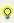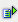# Comparison operators

Comparison operators serve for the direct comparison of two numeric values. The result of this comparison is a binary value. '0' if the condition is not fulfilled and '1' if the condition is fulfilled.Info The same comparison operators can also be used in formulas of the mathematics driver.

The following comparison operators are supported in formulas of the Combined element and the mathematics driver:

 < smaller > greater <= Lower or equal >= Higher or equal = Equal <> unequal

The following has to be cared of:

to the left and to the right of the comparison operator there has to be a (total) value or a (total) status, single bits cannot be used with these comparison operators.

To the right of the comparison operator there can also be a constant. (the constants can only be integers, a comparison to a floating point number is not possible.) In the combi element these constants additionally have to be entered as hex coded value (e.g. 0x64 is the numeric value 100).Example X01.value >= X02.value The result is 1, if the value of X01 is higher than or equal to the value of X02 X01.value = 0x64 The result is 1, if the value of X01 is exactly equal to the numeric value 100 (= hex 0x64) (X01.value = 0x64) OR (X01.value = 0x65) The result is 1, if the value of X01 is exactly equal to the numeric value 100 or 101 (= hex 0x64 and hex 0x65)